Home > GC > Chapter 8 > Lesson 8.1.3 > Problem8-27

8-27.
1. Find the area and perimeter of each shape below. Show all work. Homework Help ✎

1.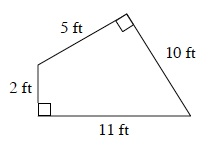2.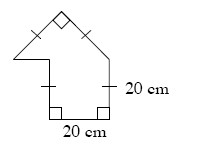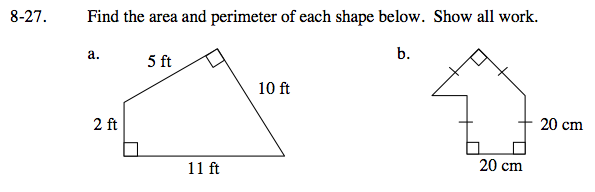How can you divide this into easier shapes to find the area?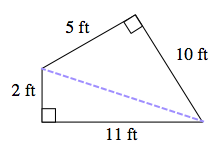See extra segment above.

Area = 36 ft2. Remember to calculate the perimeter as well.

How can you use the given values to find the length of the dashed segments?
Remember to divide the figure into easier shapes, this is a composite figure.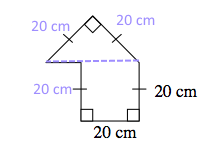Area = 600 cm², Remember to calculate the perimeter as well.